Courses

# NCERT Exemplar (Part - 1) - Solutions JEE Notes | EduRev

## JEE : NCERT Exemplar (Part - 1) - Solutions JEE Notes | EduRev

The document NCERT Exemplar (Part - 1) - Solutions JEE Notes | EduRev is a part of the JEE Course Chemistry Class 12.
All you need of JEE at this link: JEE

MULTIPLE CHOICE QUESTIONS - I

Q.1. Which of the following units is useful in relating concentration of solution with its vapour pressure?
(a) Mole fraction
(b) Parts per million
(c) Mass percentage
(d) Molality
Ans. (a)
Solution.
Mole fraction is useful in relating vapour pressure with concentration of solution. According to Raoult’s law, the partial vapour pressure of each component in the solution is directly proportional to its mole fraction.
A is one component.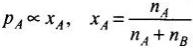Q.2. On dissolving sugar in water at room temperature solution feels cool to touch.
Under which of the following cases dissolution of sugar will be most rapid?
(a) Sugar crystals in cold water.
(b) Sugar crystals in hot water.
(c) Powdered sugar in cold water.
(d) Powdered sugar in hot water.

Ans. (d)
Solution.

Dissolution of sugar in water will be most rapid when powdered sugar is dissolved in hot water because powder form can easily insert in the vacancies of liquid particles. Further dissolution of sugar in water is an endothermic process. Hence, high temperature will favour the dissolution of sugar in water.

Q.3. At equilibrium the rate of dissolution of a solid solute in a volatile liquid  solvent is ______.
(a) Less than the rate of crystallisation
(b) Greater than the rate of crystallisation
(c) Equal to the rate of crystallisation
(d) Zero
Ans. (c)
Solution.

At equilibrium the rate of dissolution of solid in a volatile liquid solvent is equal to the rate of crystallisation.

Q.4. A beaker contains a solution of substance ‘A’. Precipitation of substance ‘A’ takes place when small amount of ‘A’ is added to the solution. The solution is ______.
(a) Saturated
(b) Supersaturated
(c) Unsaturated
(d) Concentrated

Ans. (b)
Solution.

When solute is added to the solution three cases may arise
(a) It dissolves in solution then solution is unsaturated.
(b) It does not dissolve in the solution then solution is known as saturated.
(c) When solute gets precipitated solution is known as supersaturated solution.

Q.5. Maximum amount of a solid solute that can be dissolved in a specified amount of a given liquid solvent does not depend upon ______.
(a) Temperature
(b) Nature of solute
(c) Pressure
(d) Nature of solvent
Ans. (c)
Solution.

Maximum amount of solid that can be dissolved in a specified amount of a given solvent does not depend upon pressure. This is because solid and liquid are highly incompressible and practically remain unaffected by change in pressure.

Q.6. Low concentration of oxygen in the blood and tissues of people living at high altitude is due to ______.
(a) Low temperature
(b) Low atmospheric pressure
(c) High atmospheric pressure
(d) Both low temperature and high atmospheric pressure
Ans. (b)
Solution.

Low concentration of oxygen in the blood and tissues of people living at high altitude is due to low atmospheric pressure. Because at high altitude, the partial, pressure of oxygen is less than at the ground level, so the decreased atmospheric pressure causes release of oxygen from blood.

Q.7. Considering the formation, breaking and strength of hydrogen bond, predict which of the following  mixtures will show a positive deviation from Raoult’s law?
(a) Methanol and acetone.
(b) Chloroform and acetone.
(c) Nitric acid and water.
(d) Phenol and aniline.
Ans. (a)
Solution.

In pure methanol, molecules are hydrogen bonded. On adding acetone, its molecules get in between the host molecules and break some of the hydrogen bonds between them.
Therefore, the intermolecular attractive forces between the solute-solvent molecules are weaker than those between the solute-solute and solvent-solvent molecules.
On the other hand, other three remaining options will show negative deviation from Raoult's law where the intermolecular attractive forces between the solute-solvent molecules are stronger than those between the solute-solute and solvent-solvent molecules.

Q.8. Colligative properties depend on ______.
(a) The nature of the solute particles dissolved in solution.
(b) The number of solute particles in solution.
(c) The physical properties of the solute particles dissolved in solution.
(d) The nature of solvent particles.
Ans. (b)
Solution.

Colligative properties depend upon number of solute particles in solution irrespective of their nature.

Q.9. Which of the following aqueous solutions should have the highest boiling point?
(a) 1.0 M NaOH
(b) 1.0 M Na2SO4
(c) 1.0 M NH4NO3
(d) 1.0 M KNO3
Ans. (b)
Solution.

As we know greater the value of van't Hoff factor higher will be the elevation in boiling point and hence higher will be the boiling point of solution.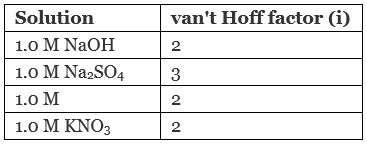Hence, 1.0 M Na2SO4 has highest value of boiling point.

Q.10. The unit of ebullioscopic constant is ______.
(a) K kg mol–1 or K (molality)–1
(b) mol kg K–1 or K–1(molality)
(c) kg mol–1 K–1 or K–1(molality)–1
(d) K mol kg–1 or K (molality)
Ans. (a)
Solution.

As we know from elevation in boiling point is given as:
ΔTb = Kbm
Kb = ΔTb/m
Where Kb = ebullioscopic constant
Thus, unit of K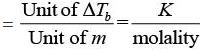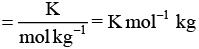Q.11. In comparison to a 0.01 M solution of glucose, the depression in freezing point of  a 0.01 M MgCl2 solution is ______.
(a) The same
Ans. (c)
Solution.

As we know depression in freezing point is directly related to van't Hoff factor (i) according to which greater the value of i greater will be the depression in freezing point.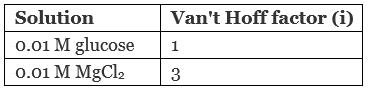Hence, depression in freezing point of glucose is about 3 times of glucose.

Q.12. An unripe mango placed in a concentrated salt solution to prepare pickle, shrivels because ______.
(a) It gains water due to osmosis.
(b) It loses water due to reverse osmosis.
(c) It gains water due to reverse osmosis.
(d) It loses water due to osmosis.
Ans. (d)
Solution.

When an unripe mango is placed in a concentrated salt solution to prepare pickle then mango loose water due to osmosis and get shrivel.

Q.13. At a given temperature, osmotic pressure of a concentrated solution of a substance ______.
(a) Is higher than that at a dilute solution.
(b) Is lower than that of a dilute solution.
(c) Is same as that of a dilute solution.
(d) Cannot be compared with osmotic pressure of dilute solution.
Ans. (a)
Solution.

According to definition of osmotic pressure we know that π = CRT. For concentrated solution C has higher value than dilute solution.
Hence, as concentration of solution increases osmotic pressure will also increase.

Q.14. Which of the following statements is false?
(a) Two different solutions of sucrose of same molality prepared in different solvents will have the same depression in freezing point.
(b) The osmotic pressure of a solution is given  by the equation π = CRT (where C is the molarity of the solution).
(c) Decreasing order of osmotic pressure for 0.01 M aqueous solutions of barium chloride, potassium chloride, acetic acid and sucrose is BaCl2 > KCl > CH3COOH > sucrose.
(d) According to Raoult’s law, the vapour pressure exerted by a volatile component of a solution is directly proportional to its mole fraction in the solution.
Ans. (a)
Solution.

Two different solutions of sucrose of same molality prepared in different solvents will have the same depression in freezing point.
According to definition of depression in freezing point.
ΔT= Kfm
Where, Kf = freezing point depression constant, value of Kf depends upon nature of solvent. This is why although the solutions have same molality two different solutions of sucrose of same molality prepared in different solvents will have different depression in freezing point.

Q.15. The values of Van’t Hoff factors for KCl, NaCl and K2SO4, respectively, are ______.
(a) 2, 2 and 2
(b) 2, 2 and 3
(c) 1, 1 and 2
(d) 1, 1 and 1

Ans. (b)
Solution.

Number of total ions present in the solution is known as van't Hoff factors (i).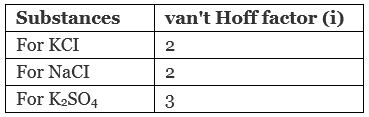Hence, correct choice is (ii).

Q.16. Which of the following statements is false?
(a) Units of atmospheric pressure and osmotic pressure are the same.
(b) In reverse osmosis, solvent molecules move through a semipermeable membrane from a region of lower concentration of solute to a region of higher concentration.
(c) The value of molal depression constant depends on nature of solvent.
(d) Relative lowering of vapour pressure, is a dimensionless quantity.
Ans. (b)
Solution.

In reverse osmosis, solvent molecules move through a semipermeable membrane from a region of higher concentration of solute to lower concentration.

Q.17. Value of Henry’s constant KH ______.
(a) Increases with increase in temperature.
(b) Decreases with increase in temperature.
(c) Remains constant.
(d) First increases then decreases.
Ans. (a)
Solution.

Value of Henry's constant (KH) increases with increase in temperature representing the decrease in solubility.

Q.18. The value of Henry’s constant KH is ______.
(a) Greater for gases with higher solubility.
(b) Greater for gases with lower solubility.
(c) Constant for all gases.
(d) Not related to the solubility of gases.
Ans. (b)
Solution.

According to Henry's law
p ∝ x
⇒ p = KHx
As value of KH rises solubility of gases decreases.

Q.19. Consider the Figure and mark the correct option.
(a) Water will move from side (A) to side (B) if a pressure lower than osmotic pressure is applied on piston (B).
(b) Water will move from side (B) to side (A) if a pressure greater than osmotic pressure is applied on piston (B).
(c) Water will move from side (B) to side (A) if a pressure equal to osmotic pressure is applied on piston (B).
(d) Water will move from side (A) to side (B) if pressure equal to osmotic pressure is applied on piston (A).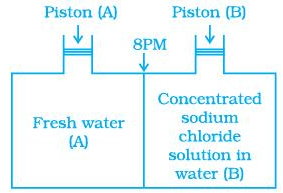Ans. (b)
Solution.
We know that, if a pressure higher than the osmotic pressure is applied on the solution. the solvent will flow from the solution into the pure solvent through the semi-permeable membrane. This process is called reverse osmosis.
Thus, in this case, water will move from side (B) to side (A) if a pressure greater than osmotic pressure is applied on piston (B).

Q.20. We have three aqueous solutions of NaCl labelled as ‘A’, ‘B’ and ‘C’ with concentrations 0.1M, 0.01M and 0.001M, respectively. The value of van’t Hoff factor for these solutions will be in the order ______.
(a) iA < iB < iC
(b) iA > iB > iC
(c) iA = iB = iC
(d) iA < iB > iC

Ans. (b)
Solution.

Van't Hoff factor is the measurement of total number of ions present in the solution. Therefore, greater the concentration of solution greater will be its van't Hoff factor.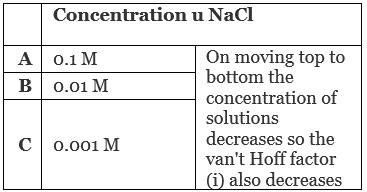Q.21. On the basis of information given below mark the correct option.
Information:
(i) In bromoethane and chloroethane mixture intermolecular interactions of A–A and B–B type are nearly same as A–B type interactions.
(ii) In ethanol and acetone mixture A–A or B–B type intermolecular interactions are stronger than A–B type interactions.
(iii) In chloroform and acetone mixture A–A or B–B type intermolecular interactions are weaker than A–B type interactions.
(a) Solution (B) and (C) will follow Raoult’s law.
(b) Solution (A) will follow Raoult’s law.
(c) Solution (B) will show negative deviation from Raoult’s law.
(d) Solution (C) will show positive deviation from Raoult’s law.

Ans. (b)
Solution.

For an ideal solution, the A—A or B—B type intermolecular interaction is nearly equal to A—B type interaction. Here, a mixture of bromoethane and chloroethane is an example of ideal solution.
On the other hand chloroform and acetone mixture is an example of non-ideal solution having negative deviation. So, (A — A) or (B—B) interaction must be stronger than A—B interaction. While ethanol-acetone mixture shows positive deviation due to weaker A—B interaction in comparison to A—A or A—B interaction.

Q.22. Two beakers of capacity 500 mL were taken. One of these beakers, labelled as “A”, was filled with 400 mL water whereas the beaker labelled “B” was filled with 400 mL of 2M solution of NaCl. At the same temperature both the beakers were placed in closed containers of same material and same capacity as shown in Figure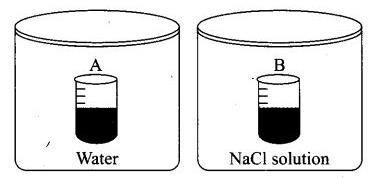At a given temperature, which of the following statement is correct about the vapour pressure of pure water and that of NaCl solution.
(a) Vapour pressure in container (A) is more than that in container (B).
(b) Vapour pressure in container (A) is less than that in container (B).
(c) Vapour pressure is equal in both the containers.
(d) Vapour pressure in container (B) is twice the vapour pressure in container (A).

Ans. (a)
Solution.

When salt is added to water to make the solution, the vapour pressure of solution decreases. This is due to decrease in surface covered by solvent molecule which leads to decrease in number of solvent molecule escaping from the surface corresponding to pure solvent.
Hence, vapour pressure also get reduces.

Q.23. If two liquids A and B form minimum boiling azeotrope at some specific composition then ________.
(a) A–B interactions are stronger than those between A–A or B–B.
(b) Vapour pressure of solution increases because more number of molecules of liquids A and B can escape from the solution.
(c) Vapour pressure of solution decreases because less number of molecules of only one of the liquids escape from the solution.
(d) A–B interactions are weaker than those between A–A or B–B.
Ans. (a)
Solution.

If two liquids A and B form minimum boiling azeotrope at some specific composition then A—B interactions are weaker than those of A—A and B—B. It is due to the fact that in case of positive deviation, we get minimum boiling azeotropes whereas in case of negative deviation we get maximum boiling azeotropes.

Q.24. 4L of 0.02 M aqueous solution of NaCl was diluted by adding one litre of water. The molality of the resultant solution is ______.
(a) 0.004
(b) 0.008
(c) 0.012
(d) 0.016

Ans. (d)
Solution.

Given, M1 = 0.02M, V1 = 4L, M2 = ?, V2 = 4L + 1L = 5L
As we know, M1V1 = M2V2
Where, V1 = volume of solution before dilution
V2 = volume of solution after dilution
M= strength of solution before dilution
M2 = strength of solution after dilution
0.02 × 4L = M2 × 5L
M2 = 0.08/5 = 0.016 M

Q.25. On the basis of information given below mark the correct option.
Information: On adding acetone to methanol some of the hydrogen bonds between methanol molecules break.
(a) At specific composition methanol-acetone mixture will form minimum boiling azeotrope and will show positive deviation from Raoult’s law.
(b) At specific composition methanol-acetone mixture will forms maximum boiling azeotrope and will show positive deviation from Raoult’s law.
(c) At specific composition methanol-acetone mixture will form minimum boiling azeotrope and will show negative deviation from Raoult’s law.
(d) At specific composition methanol-acetone mixture will form maximum boiling azeotrope and will show negative deviation from Raoult’s law.

Ans. (a)
Solution.

At specific composition methanol- acetone mixture will form minimum boiling azeotrope and will show positive deviation. This is due to weaker A—B interaction than A—A or B—B interaction. i.e., A—B < A—A and B—B

Q.26. KH value for Ar(g), CO2(g), HCHO (g) and CH4(g) are 40.39, 1.67, 1.83×10–5 and 0.413 respectively.
Arrange these gases in the order of their increasing solubility.
(a) HCHO < CH4  < CO2 < Ar
(b) HCHO < CO2 < CH4 < Ar
(c) Ar < CO2 < CH4 < HCHO
(d) Ar < CH< CO2 < HCHO

Ans. (c)
Solution.

We know that higher the value of KH at a given pressure, the lower is the solubility of the gas in the liquid.
Now, value of KH depends upon nature of gases dissolved in water.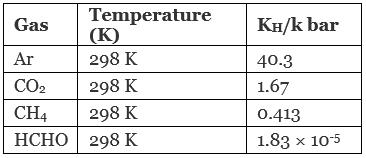Hence, correct order is Ar < CO2 < CH4 < HCHO and correct choice is (iii).

MULTIPLE CHOICE QUESTIONS - II

Note: In the following questions two or more options may be correct.
Q.27. Which of the following factor (s) affect the solubility of a gaseous solute in the fixed volume of liquid solvent?
(a) Nature of solute
(b) Temperature
(c) Pressure
(i) (a) and (c) at constant T
(ii) (a) and (b) at constant P
(iii) (b) and (c) only
(iv) (c) only

Ans. (i, ii)
Solution.

Solubility of gaseous solute in the fixed volume of liquid solvent always depends upon nature of solute. It also depends upon pressure at constant temperature and temperature at constant pressure.

Q.28. Intermolecular forces between two benzene molecules are nearly of same strength as those between two toluene molecules. For a mixture of benzene and toluene, which of the following are not true?
(a) ∆mix H = zero
(b) ∆mix V = zero
(c) These will form minimum boiling azeotrope.
(d) These will not form ideal solution.
Ans. (c, d)
Solution.

The solution which follows Raoult's law is known as ideal solution. For an ideal solution intermolecular forces between two benzene molecules are nearly of same strength as those between two toluene molecules. For an ideal solution
∆Vmix = 0 and ∆Hmix = 0
Thus, the mixture of benzene and toluene is an example of ideal solution. Option (iii) is incorrect as minimum boiling azeotropes are formed by non-ideal solution.

Q.29. Relative lowering of vapour pressure is a colligative property because ______.
(a) It depends on the concentration of a non electrolyte solute in solution and does not depend on the nature of the solute molecules.
(b) It depends on number of particles of electrolyte solute in solution and does not depend on the nature of the solute particles.
(c) It depends on the concentration of a non electrolyte solute in solution as well as on the nature of the solute molecules.
(d) It depends on the concentration of an electrolyte or nonelectrolyte solute in  solution as well as on the nature of solute molecules.

Ans. (a, b)
Solution.

Relative lowering of vapour pressure is a Colligative property because
(a) It does not depend upon nature of solute.
(b) It depends upon number of solute particles.
(c) It depends upon concentration of non-electrolyte solution.

Q.30. Van’t Hoff factor (i) is given by the expression ______.
(a)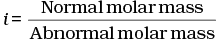(b)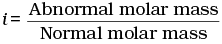(c)(d)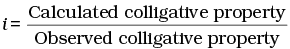Ans. (a, c)
Solution.

Van't Hoff factor (i) is a measure of extent of association or dissociation of solute particles which can be calculated as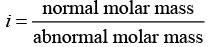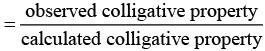Q.31. Isotonic solutions must have the same ______.
(a) Solute
(b) Density
(c) Elevation in boiling point
(d) Depression in freezing point
Ans. (c, d)
Solution.

Isotonic solutions have same osmotic pressure and same concentration. Elevation in boiling point and depression in freezing point are the Colligative properties. These two Colligative properties depend upon concentration.
As the molar concentration is same for isotonic solutions, so elevation in boiling point and depression in freezing point of isotonic solutions must be same.

Q.32. Which of the following binary mixtures will have same composition in liquid and vapour phase?
(a) Benzene - Toluene
(b) Water -Nitric acid
(c) Water -Ethanol
(d) n-Hexane - n-Heptane
Ans. (b, c)
Solution.

Mixtures having same composition in liquid and vapour phase are known as azeotropes. Azeotrope boils at same temperature.
Here, water-nitric acid and water-ethanol mixtures are non-ideal solution. Hence, water-nitric acid and water-ethanol are examples of azeotropes.
While benzene-toluene and n-hexane-n -heptane are examples of ideal solution.

Q.33. In isotonic solutions ______.
(a) Solute and solvent both are same.
(b) Osmotic pressure is same.
(c) Solute and solvent may or may not be same.
(d) Solute is always same solvent may be different.
Ans. (b, c)
Solution.

The two solutions having same osmotic pressure are known as isotonic solutions. The solute and solvent particles may or may not be same but osmotic pressure must be same.

Q.34. For a binary ideal liquid solution, the variation in total vapour pressure versus composition of solution is given by which of the curves?
(a)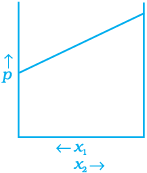(b)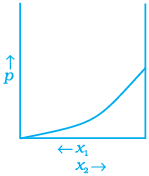(c)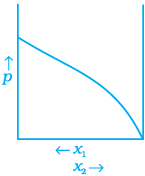(c)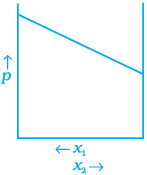Ans. (a, d)
Solution.
Depending on the vapour pressures of the pure components 1 and 2, total vapour pressure over the solution decreases or increases with the increase of the mole fraction of component 1.

Q.35. Colligative properties are observed when ______.
(a) A non volatile solid is dissolved in a volatile liquid.
(b) A non volatile liquid is dissolved in another volatile liquid.
(c) A gas is dissolved in non volatile liquid.
(d) A volatile liquid is dissolved in another volatile liquid.

Ans. (a, b)
Solution.

When any of one components of binary mixture either solvent or solute is volatile it causes deviation from ideal behaviour and vapour pressure of solution which causes change in Colligative property.

Offer running on EduRev: Apply code STAYHOME200 to get INR 200 off on our premium plan EduRev Infinity!

## Chemistry Class 12

255 videos|306 docs|201 tests

,

,

,

,

,

,

,

,

,

,

,

,

,

,

,

,

,

,

,

,

,

;